Worksheet Solution: How Many Squares?

# Worksheet Solution: How Many Squares? Notes | Study Mathematics for Class 5: NCERT - Class 5

## Document Description: Worksheet Solution: How Many Squares? for Class 5 2022 is part of Mathematics for Class 5: NCERT preparation. The notes and questions for Worksheet Solution: How Many Squares? have been prepared according to the Class 5 exam syllabus. Information about Worksheet Solution: How Many Squares? covers topics like and Worksheet Solution: How Many Squares? Example, for Class 5 2022 Exam. Find important definitions, questions, notes, meanings, examples, exercises and tests below for Worksheet Solution: How Many Squares?.

Introduction of Worksheet Solution: How Many Squares? in English is available as part of our Mathematics for Class 5: NCERT for Class 5 & Worksheet Solution: How Many Squares? in Hindi for Mathematics for Class 5: NCERT course. Download more important topics related with notes, lectures and mock test series for Class 5 Exam by signing up for free. Class 5: Worksheet Solution: How Many Squares? Notes | Study Mathematics for Class 5: NCERT - Class 5
 1 Crore+ students have signed up on EduRev. Have you?

Q.1. Draw 2 straight lines in this rectangle to divide it into 1 rectangle and 2 equal triangles. Find the area of the new rectangle and the area of 1 of the triangles.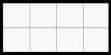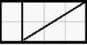Q.2. Write the area in square cm of the shaded portion.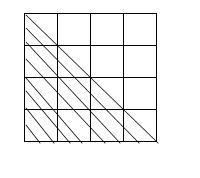Total number of squares in the given figure = 16
The shaded portion is half of the given figure
So the area of the shaded portion = 8 square cm

Q.3. Find the perimeter and area of the following figure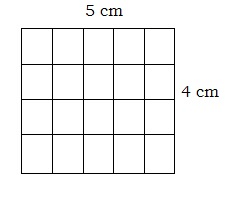The perimeter is the length of the boundary.
So perimeter = 18 cm
Area = Total number of squares = 20 square cm

Q.4.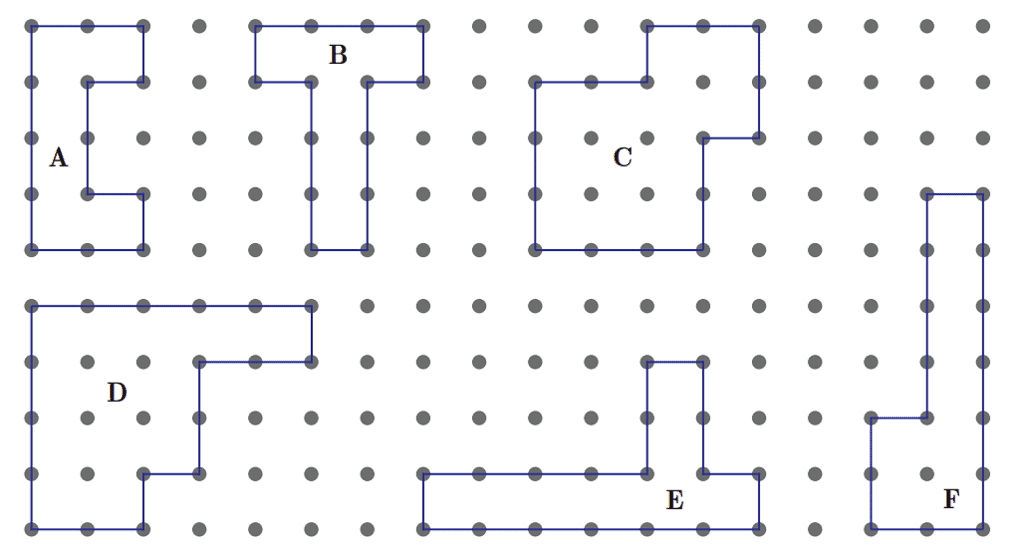(a)  Which shape has the maximum perimeter? Its perimeter is?

Shape D has the maximum perimeter
Its perimeter is 18 cm

(b) Which shape has the minimum perimeter? Its perimeter is

Shape A has the minimum perimeter
Its perimeter is 14 cm

(c) The perimeter of figure D is____cm. It is _____ (less/more) than the perimeter of figure A which is______ cm

The perimeter of D is 18 cm.
It is more than the perimeter of A, which is 14 cm.

Q.5. Study the diagram given below. Draw 2 more lines so that the area is 18 square cm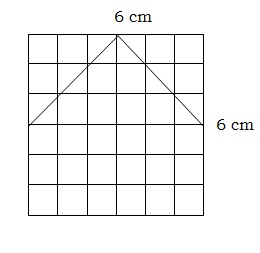Consider the given square as four equal squares.
The first line is half of the first square.
So its area is 4.5 square cm
Area of the second square also 4.5 square cm
So total area is 9 square cm
So to get 18 square cm area we can draw two lines as follows: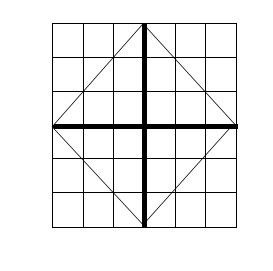Q.6. Here is a rectangle of an area of 20 square cm.
a). Draw one straight line in this rectangle to divide it into two equal triangles. What is the area of each of the triangles?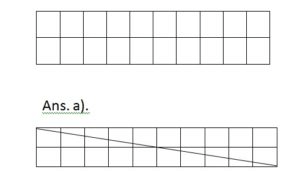Area of each triangle = ½ X area of a rectangle
= ½ X 20 = 10 square cm

b) Draw one straight line in this rectangle to divide it into two equal rectangles. What is the area of each of the smaller rectangles?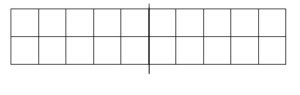Area of each smaller rectangle = ½ area of the bigger rectangle
= ½ X 20 = 10 square cm

c) Draw two straight lines in this rectangle to divide it into one rectangle and two equal triangles. What is the area of the rectangle? What is the area of each of the triangles?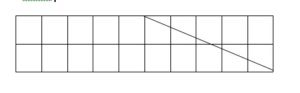Area of rectangle = 10 square cm
Area of each triangle = ½ x area of the smaller rectangle
= ½ X 10 = 5 square cm

Q.7. Find the perimeter and area of each rectangle.
(a)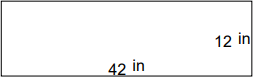Perimeter = length + breadth + length + breadth

Perimeter = 42 + 12 + 42 + 12

Perimeter = 108 in

Area = length x breadth

Area = 42 x 12

Area = 504 in²

(b)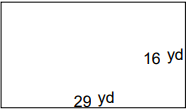Perimeter = length + breadth + length + breadth

Perimeter = 29 + 16 + 29 + 16

Perimeter = 90 yd

Area = length x breadth

Area = 29 x 16

Area = 464 yd²

(c)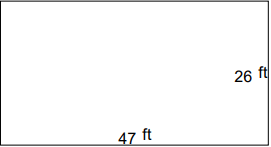Perimeter = length + breadth + length + breadth

Perimeter =47 + 26 + 47 + 26

Perimeter = 146 ft

Area = length x breadth

Area = 47 x 26

Area = 1,222 ft²

(d)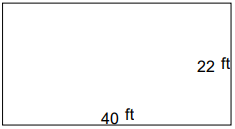Perimeter = length + breadth + length + breadth

Perimeter = 40 + 22 + 40 + 22

Perimeter = 124 ft

Area = length x breadth

Area = 40 x 22

Area = 880 ft²

(e)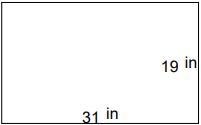Perimeter = length + breadth + length + breadth

Perimeter = 31 + 19 + 31 + 19

Perimeter = 100 in

Area = length x breadth

Area = 31 x 19

Area = 589 in²

(f)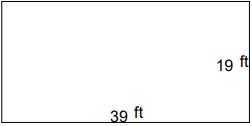Perimeter = length + breadth + length + breadth

Perimeter = 39 + 19 + 39 + 19

Perimeter = 116 ft

Area = length x breadth

Area = 39 x 19

Area =741 ft²

(g)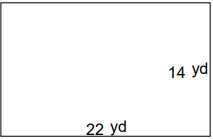Perimeter = length + breadth + length + breadth

Perimeter = 22 + 14 + 22 + 14

Perimeter = 72 yd

Area = length x breadth

Area =  22 x 14

Area = 308 yd²

(h)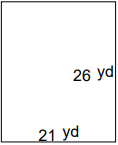Perimeter = length + breadth + length + breadth

Perimeter = 21 + 26 + 21 + 26

Perimeter = 94 yd

Area = length x breadth

Area = 21 x 26

Area = 546 yd²

(i)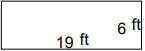Perimeter = length + breadth + length + breadth

Perimeter = 19 + 6 + 19 + 6

Perimeter = 50 ft

Area = length x breadth

Area = 19 x 6

Area = 114 ft²

(j)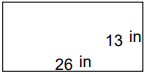Perimeter = length + breadth + length + breadth

Perimeter = 26 + 13 + 26 + 23

Perimeter = 78 in

Area = length x breadth

Area = 26 x 13

Area = 338 in²

Q.8.
(a) The area of a square is 9 cm. Find the area of shaded part: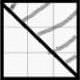Area of the square = 9 cm

The shaded part is half of the square
So the area of the shaded part = Area of square/ 2

9/2 = 4.5 sq. cm

(b) Find the perimeter of the following figure: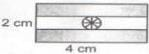Perimeter = Sum of all sides

So, 2+4+2+4 = 12 cm

(c) Find the area of the shaded shape: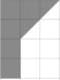The shaded shape includes 5 squares and 2 triangles
Area of 1 square = 1 cm
Area of triangle = Area of square/2
Area of 2 triangles = 1/2 + 1/2 = 1

Total area = 5 +1 = 6 sq. cm

(d) Find the area of the following: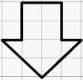The shaded shape includes 6 squares and 4 triangles

Area of 1 square = 1 cm

Area of 6 squares = 6 cm

Area of triangle = Area of square/2

Area of 4 triangles = 1/2 + 1/2 + 1/2 + 1/2 = 2

Total area = 6 +2 = 8 sq.cm

(e) Find the area of the shaded path.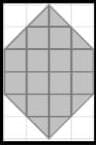The shaded path includes 12 squares and 8 triangles

Area of 1 square = 1 cm

Area of 12 squares = 12 cm

Area of triangle = Area of square/2

Area of 8 triangles = 1/2 + 1/2 + 1/2 + 1/2 + 1/2 + 1/2 + 1/2 + 1/2 = 4

Total area = 12 +4 = 16 sq.cm

The document Worksheet Solution: How Many Squares? Notes | Study Mathematics for Class 5: NCERT - Class 5 is a part of the Class 5 Course Mathematics for Class 5: NCERT.
All you need of Class 5 at this link: Class 5

## Mathematics for Class 5: NCERT

33 videos|59 docs|39 tests
 Use Code STAYHOME200 and get INR 200 additional OFF

## Mathematics for Class 5: NCERT

33 videos|59 docs|39 tests

Track your progress, build streaks, highlight & save important lessons and more!

,

,

,

,

,

,

,

,

,

,

,

,

,

,

,

,

,

,

,

,

,

;